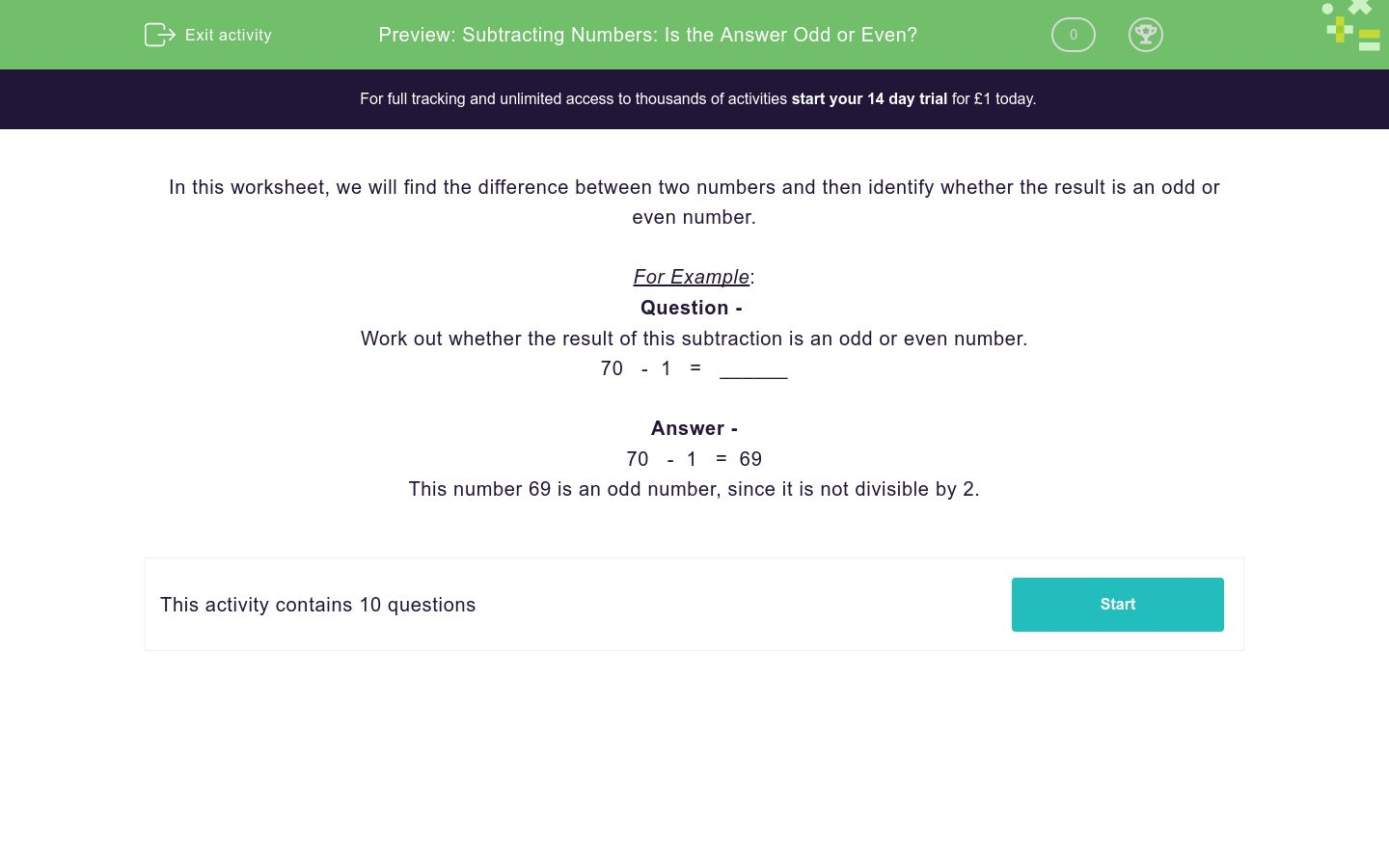# Subtracting Numbers: Is the Answer Odd or Even?

In this worksheet, students subtract one number from another and identify if the answer is an odd or even number.Key stage:  KS 2

Curriculum topic:  Number: Addition and Subtraction

Curriculum subtopic:  Add/Subtract up to Four-Digit Numbers

Difficulty level:### QUESTION 1 of 10

In this worksheet, we will find the difference between two numbers and then identify whether the result is an odd or even number.

For Example:

Question -
Work out whether the result of this subtraction is an odd or even number.

70   -  1   =   ______

Answer -

70   -  1   =  69

This number 69 is an odd number, since it is not divisible by 2.

Work out whether the result of this subtraction is an odd or even number.

55   -  34   =   ______

21 (Odd)

21 (Even)

18 (Odd)

18 (Even)

Work out whether the result of this subtraction is an odd or even number.

60   -  7   =   ______

51 (Odd)

51 (Even)

53 (Odd)

53 (Even)

Work out whether the result of this subtraction is an odd or even number.

72   -  67   =   ______

5 (Even)

5 (Odd)

7 (Odd)

7 (Even)

Work out whether the result of this subtraction is an odd or even number.

48   -  16   =   ______

33 (Even)

33 (Odd)

32 (Odd)

32 (Even)

Work out whether the result of this subtraction is an odd or even number.

66   -  26   =   ______

40 (Even)

40 (Odd)

50 (Even)

50 (Odd)

Work out whether the result of this subtraction is an odd or even number.

52   -  47   =   ______

5 (Even)

5 (Odd)

3 (Even)

3 (Odd)

Work out whether the result of this subtraction is an odd or even number.

151   -  50   =   ______

101 (Even)

101 (Odd)

11 (Even)

11 (Odd)

When you subtract an odd number from an odd number, what can you say about the result?

Odd

Even

Can't be sure

When you subtract an odd number from an even number, what can you say about the result?

Odd

Even

Can't be sure

• Question 1

Work out whether the result of this subtraction is an odd or even number.

55   -  34   =   ______

CORRECT ANSWER
21 (Odd)
• Question 2

Work out whether the result of this subtraction is an odd or even number.

60   -  7   =   ______

CORRECT ANSWER
53 (Odd)
• Question 3

Work out whether the result of this subtraction is an odd or even number.

72   -  67   =   ______

CORRECT ANSWER
5 (Odd)
• Question 4

Work out whether the result of this subtraction is an odd or even number.

48   -  16   =   ______

CORRECT ANSWER
32 (Even)
• Question 5

Work out whether the result of this subtraction is an odd or even number.

66   -  26   =   ______

CORRECT ANSWER
40 (Even)
• Question 6

Work out whether the result of this subtraction is an odd or even number.

52   -  47   =   ______

CORRECT ANSWER
5 (Odd)
• Question 7

Work out whether the result of this subtraction is an odd or even number.

151   -  50   =   ______

CORRECT ANSWER
101 (Odd)
• Question 8

When you subtract an odd number from an odd number, what can you say about the result?

CORRECT ANSWER
Even
• Question 9

When you subtract an odd number from an even number, what can you say about the result?

CORRECT ANSWER
Odd
• Question 10
---- OR ----

Sign up for a £1 trial so you can track and measure your child's progress on this activity.

### What is EdPlace?

We're your National Curriculum aligned online education content provider helping each child succeed in English, maths and science from year 1 to GCSE. With an EdPlace account you’ll be able to track and measure progress, helping each child achieve their best. We build confidence and attainment by personalising each child’s learning at a level that suits them.

Get startedStart your £1 trial today.
Subscribe from £10/month.